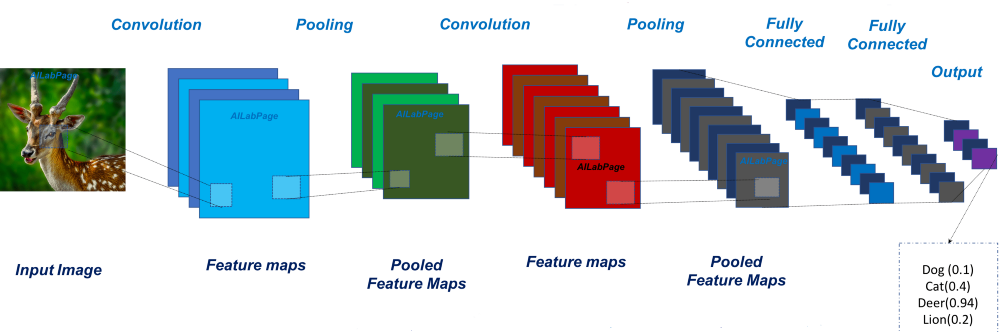# Simple Convolutional Neural Network Using Keras

Original article was published on Deep Learning on Medium# Simple Convolutional Neural Network Using Keras

Convolutional neural network is a class of deep neural networks. It’s called deep because it has many layers on its architecture. CNN is commonly used for analyzing visual imagery.

CNN consists of input, hidden layer and output layer. The hidden layer typically consists of a series of convolutions layers, pooling layer, normalization layer, etc.

In this article we will use Keras for creating the architecture and running the computation. Keras is a python library that help us to build neural net pretty simple and easy.

We will try to build model for classifying MNIST dataset(28×28 images), which consists of 70,000 handwritten images from 0–9.

## Preparing the data

Keras library prepared the MNIST dataset for us to use.

`from keras.datasets import mnist`

After import the dataset, we need to load the dataset into training datasets and testing datasets. The MNIST data gave us 60,000 data for training and 10,000 data for testing

`(x_train, y_train), (x_test, y_test) = mnist.load_data()print('Training data count: {}'.format(x_train.shape))print('Testing data count: {}'.format(x_test.shape))`

The MNIST data will look like this:

## Data preprocessing

After loading the data, we need to pre-process our data before feeding the data into the network. We know that MNIST data is 28×28 images, and the model will expects input with shape (data_count, weight, height, channel). So, we need to reshape our data and the code will look like this:

`x_train = x_train.reshape(60000,28,28,1)x_test = x_test.reshape(10000,28,28,1)`

After that, we should pre-process the label of each data using one-hot encoder. This creates a binary column for each category and returns a sparse matrix or dense array.

There are many ways to encode the label, this code is using sklearn library:

`from sklearn.preprocessing import OneHotEncoderencoder = OneHotEncoder(sparse=False)y_train = y_train.reshape(-1, 1)y_train = encoder.fit_transform(y_train)y_test= y_train.reshape(-1, 1)y_test = encoder.fit_transform(y_test)`

This one is using Keras

`from keras.utils import to_categoricaly_train = to_categorical(y_train)y_test = to_categorical(y_test)`

## Building The Model

We’re ready with our data, now we build a sequential model with Keras. Why Sequential? because, sequential model is used to build models as a simple stack of layers.

`from keras.models import Sequentialfrom keras.layers import Dense, Conv2D, Flattenmodel = Sequential()model.add(Conv2D(16, kernel_size=3, activation='relu', input_shape=(28,28,1)))model.add(Conv2D(8, kernel_size=3, activation='relu'))model.add(Flatten())model.add(Dense(10, activation='softmax'))`

The 16 in first convolution layer and 8 in the second convolution layer is the number of nodes in that layer (can be adjusted), kernel_size is the size of convolution window.

After the convolution layer, there is a flatten layer. It convert the output of last convolution layer to one dimensional array.

Dense layer is the classic layer that used in many cases of neural network. we can add the other dense layer to make our network smarter (not always!).

## Compiling The Model

After creating the model, we need to compile the model. It needs optimizer, loss function and a list of metrics.

`model.compile(optimizer='adam', loss='categorical_crossentropy', metrics=['accuracy'])`

We use ‘adam’ optimizer because its pretty good (you can try another optimizer too).

The loss function we are using is categorical_crossentropy and we use softmax in the last layer because, our data is multi-class and we are making single label classification model. You can refer to this article to find the details about loss function and last layer activation.

## Training the Model

`history = model.fit(x_train, y_train, validation_data=(x_test, y_test), epochs=5)`

We simply call the fit function from our model, and Keras will automatically run the computation for training our data. As you can see in the code above, fit method need the following parameters: x_data, y_data, number of epoch. The validation data is an optional parameters. We used the validation data to check is our model good enough or maybe overfit in there.

We got 97.06% in our validation(test) dataset. That’s good enough for our model. We can tuning the hyperparameters to make our model greater.

## Using The Model to Make Predictions

We can simply pass some array of input to the predict method

`prediction = model.predict(x_test[:3]) #first 3 data of test data`

and it will returns an array of output which looks like this:

We can get the actual digit using argmax function from numpy. below code is plotting the test image with the label:

`import numpy as npprediction = model.predict(x_test[:3])print(prediction)w=60h=40fig=plt.figure(figsize=(15, 15))columns = 3rows = 1for i in range(1, columns*rows +1): ax = fig.add_subplot(rows, columns, i) plt.xticks([], []) plt.yticks([], [])actual_label = np.argmax(y_test[i-1]) prediction_label = np.argmax(prediction[i-1]) ax.title.set_text('Prediction: {} - Actual: {}'.format(prediction_label, actual_label)) image = x_test[i-1].reshape((28,28)) plt.imshow(image, cmap='gray')plt.show()`

Finally, you have created your own model to classify MNIST data. Congrats!! 👏👏👏

I will provide the full source code on google colab or github for you reference.